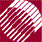ECE532 Biomedical Optics © 1998 Steven L. Jacques, Scott A. Prahl Oregon Graduate Institute

# Experiment: Calibrating a laser

We shall calibrate the radiant power, P [W], of a laser by aiming it into a cup of black water and capturing narly all the radiant energy, Q [J], generated during an exposure time, t [s]. Measuring the temperature rise of the water will yield the power of the laser.

• Start with our 500-ml cup of black water at 25 °C (Ti).

• Aim a 10-W laser into the cup of black water, perpendicular to the water surface.

• Turn on the laser for 60 s, stirring the water while the laser is on.

• Measure the final temperature rise (Tf = 25.281 °C).

• For water:
• density = rho = 1 [g/cm3]
• heat capacity = C = 4.18 J/(g °C)
• rho*C = 4.18 J/(cm3 °C)
• We shall neglect the heat capacity and mass of the cup itself in this calculation. The cup causes a change in the apparent value of the product rho*C*V. See Discussion of cup for guide to considering this additional factor in a real experiment.

• Total energy deposited in water:
Q = (Tf - Ti)*rhoC*V = (25.281 - 25)*4.18*500 = 588 J

• Power deposition in water:
P = Q/t = 588/60 = 9.8 W

• These values for Q and P are only 98% of the values for the light bulb experiment despite the light bulb and laser both delivering 10 W. The reason is that 2% of the incident laser light bounced off the air/water surface of our cup of black water. Only the light that entered the cup of black water caused heating of the water. The refractive index of water is about 1.33 and that of air is 1.0. Specular reflectance off the air/water surface equals:
rsp = ( (1.33 - 1.0)/(1.33 + 1.0) )2 = 0.02006

• Laser power:
P/0.98 = 10 W

The radiant power P of the laser is 10 W.